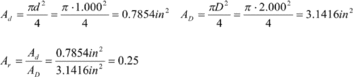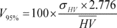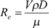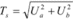# Title 43

## SECTION 3175.10

### 3175.10 Definitions and acronyms.

§ 3175.10 Definitions and acronyms.

(a) As used in this subpart, the term:

AGA Report No. (followed by a number) means a standard prescribed by the American Gas Association, with the number referring to the specific standard.

Area ratio means the smallest unrestricted area at the primary device divided by the cross-sectional area of the meter tube. For example, the area ratio (Ar) of an orifice plate is the area of the orifice bore (Ad) divided by the area of the meter tube (AD). For an orifice plate with a bore diameter (d) of 1.000 inches in a meter tube with an inside diameter (D) of 2.000 inches the area ratio is 0.25 and is calculated as follows:As-found means the reading of a mechanical or electronic transducer when compared to a certified test device, prior to making any adjustments to the transducer.

As-left means the reading of a mechanical or electronic transducer when compared to a certified test device, after making adjustments to the transducer, but prior to returning the transducer to service.

Atmospheric pressure means the pressure exerted by the weight of the atmosphere at a specific location.

Beta ratio means the measured diameter of the orifice bore divided by the measured inside diameter of the meter tube. This is also referred to as a diameter ratio.

Bias means a systematic shift in the mean value of a set of measurements away from the true value of what is being measured.

British thermal unit (Btu) means the amount of heat needed to raise the temperature of one pound of water by 1 °F.

Component-type electronic gas measurement system means an electronic gas measurement system comprising transducers and a flow computer, each identified by a separate make and model, from which performance specifications are obtained.

Configuration log means a list of all fixed or user-programmable parameters used by the flow computer that could affect the calculation or verification of flow rate, volume, or heating value.

Discharge coefficient means an empirically derived correction factor that is applied to the theoretical differential flow equation in order to calculate a flow rate that is within stated uncertainty limits.

Effective date of a spot or composite gas sample means the first day on which the relative density and heating value determined from the sample are used in calculating the volume and quality on which royalty is based.

Electronic gas measurement (EGM) means all of the hardware and software necessary to convert the static pressure, differential pressure, and flowing temperature developed as part of a primary device, to a quantity, rate, or quality measurement that is used to determine Federal royalty. For orifice meters, this includes the differential-pressure transducer, static-pressure transducer, flowing-temperature transducer, on-line gas chromatograph (if used), flow computer, display, memory, and any internal or external processes used to edit and present the data or values measured.

Element range means the difference between the minimum and maximum value that the element (differential-pressure bellows, static-pressure element, and temperature element) of a mechanical recorder is designed to measure.

Event log means an electronic record of all exceptions and changes to the flow parameters contained within the configuration log that occur and have an impact on a quantity transaction record.

GPA (followed by a number) means a standard prescribed by the Gas Processors Association, with the number referring to the specific standard.

Heating value means the gross heat energy released by the complete combustion of one standard cubic foot of gas at 14.73 pounds per square inch absolute (psia) and 60 °F.

Heating value variability means the deviation of previous heating values over a given time period from the average heating value over that same time period, calculated at a 95 percent confidence level. Unless otherwise approved by the BLM, variability is determined with the following equation:Where: V95% = heating value variability, % σHV = standard deviation of the previous 5 heating values 2.776 = the “student-t” function for a probability of 0.05 and 4 degrees of freedom (degree of freedom is the number of samples minus 1) HV= the average heating value over the time period used to determine the standard deviation

High-volume facility measurement point or high-volume FMP means any FMP that measures more than 200 Mcf/day, but less than or equal to 1,000 Mcf/day over the averaging period.

Hydrocarbon dew point means the temperature at which hydrocarbon liquids begin to form within a gas mixture. For the purpose of this regulation, the hydrocarbon dew point is the flowing temperature of the gas measured at the FMP, unless otherwise approved by the AO.

Integration means a process by which the lines on a circular chart (differential pressure, static pressure, and flowing temperature) used in conjunction with a mechanical chart recorder are re-traced or interpreted in order to determine the volume that is represented by the area under the lines. An integration statement documents the values determined from the integration.

Live input variable means a datum that is automatically obtained in real time by an EGM system.

Low-volume facility measurement point or low-volume FMP means any FMP that measures more than 35 Mcf/day, but less than or equal to 200 Mcf/day, over the averaging period.

Lower calibrated limit means the minimum engineering value for which a transducer was calibrated by certified equipment, either in the factory or in the field.

Mean means the sum of all the values in a data set divided by the number of values in the data set.

Mole percent means the number of molecules of a particular type that are present in a gas mixture divided by the total number of molecules in the gas mixture, expressed as a percentage.

Normal flowing point means the differential pressure, static pressure, and flowing temperature at which an FMP normally operates when gas is flowing through it.

Primary device means the volume-measurement equipment installed in a pipeline that creates a measureable and predictable pressure drop in response to the flow rate of fluid through the pipeline. It includes the pressure-drop device, device holder, pressure taps, required lengths of pipe upstream and downstream of the pressure-drop device, and any flow conditioners that may be used to establish a fully developed symmetrical flow profile.

Qualified test facility means a facility with currently certified measurement systems for mass, length, time, temperature, and pressure traceable to the NIST primary standards or applicable international standards approved by the BLM.

Quantity transaction record (QTR) means a report generated by an EGM system that summarizes the daily and hourly volumes calculated by the flow computer and the average or totals of the dynamic data that is used in the calculation of volume.

Reynolds number means the ratio of the inertial forces to the viscous forces of the fluid flow, and is defined as:Where: Re = the Reynolds number V = velocity ρ = fluid density D = inside meter tube diameter µ = fluid viscosity

Redundancy verification means a process of verifying the accuracy of an EGM system by comparing the readings of two sets of transducers placed on the same primary device.

Secondary device means the differential-pressure, static-pressure, and temperature transducers in an EGM system, or a mechanical recorder, including the differential pressure, static pressure, and temperature elements, and the clock, pens, pen linkages, and circular chart.

Self-contained EGM system means an EGM system in which the transducers and flow computer are identified by a single make and model number from which the performance specifications for the transducers and flow computer are obtained. Any change to the make or model numbers of either a transducer or a flow computer within a self-contained EGM system changes the system to a component-type EGM system.

Senior fitting means a type of orifice plate holder that allows the orifice plate to be removed, inspected, and replaced without isolating and depressurizing the meter tube.

Standard cubic foot (scf) means a cubic foot of gas at 14.73 psia and 60 °F.

Standard deviation means a measure of the variation in a distribution, and is equal to the square root of the arithmetic mean of the squares of the deviations of each value in the distribution from the arithmetic mean of the distribution.

Tertiary device means, for EGM systems, the flow computer and associated memory, calculation, and display functions.

Threshold of significance means the maximum difference between two data sets (a and b) that can be attributed to uncertainty effects. The threshold of significance is determined as follows:Where: Ts = Threshold of significance, in percent Ua = Uncertainty (95 percent confidence) of data set a, in percent Ub = Uncertainty (95 percent confidence) of data set b, in percent

Transducer means an electronic device that converts a physical property such as pressure, temperature, or electrical resistance into an electrical output signal that varies proportionally with the magnitude of the physical property. Typical output signals are in the form of electrical potential (volts), current (milliamps), or digital pressure or temperature readings. The term transducer includes devices commonly referred to as transmitters.

Turndown means a reduction of the measurement range of a transducer in order to improve measurement accuracy at the lower end of its scale. It is typically expressed as the ratio of the upper range limit to the upper calibrated limit.

Type test means a test on a representative number of a specific make, model, and range of a device to determine its performance over a range of operating conditions.

Uncertainty means the range of error that could occur between a measured value and the true value being measured, calculated at a 95 percent confidence level.

Upper calibrated limit means the maximum engineering value for which a transducer was calibrated by certified equipment, either in the factory or in the field.

Upper range limit (URL) means the maximum value that a transducer is designed to measure.

Verification means the process of determining the amount of error in a differential pressure, static pressure, or temperature transducer or element by comparing the readings of the transducer or element with the readings from a certified test device with known accuracy.

Very-low-volume facility measurement point or very-low-volume FMP means any FMP that measures 35 Mcf/day or less over the averaging period.

Very-high-volume facility measurement point or very-high-volume FMP means any FMP that measures more than 1,000 Mcf/day over the averaging period.

(b) As used in this subpart the following additional acronyms carry the meaning prescribed:

GARVS means the BLM's Gas Analysis Reporting and Verification System.

GC means gas chromatograph.

GPA means the Gas Processors Association.

Mcf means 1,000 standard cubic feet.

psia means pounds per square inch - absolute.

psig means pounds per square inch - gauge.